# NCERT Solutions For Class 6 Maths Symmetry Exercise 13.2

NCERT Solutions For Class 6 Maths Symmetry Exercise 13.2

### NCERT Solutions For Class 6 Maths Symmetry Exercise 13.2

NCERT Solutions For Class 6 Maths Chapter 13 Symmetry Ex 13.2

Exercise 13.2

Ex 13.2 Class 6 Maths Question 1.
Find the number of lines of symmetry for each of the following shapes.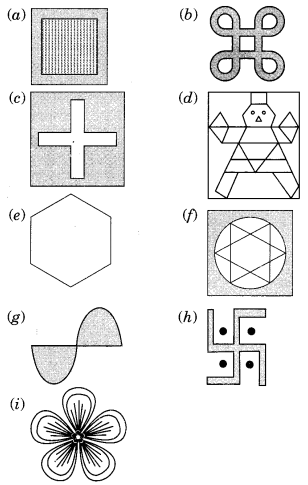Solution:
(a) Here, there are four symmetric lines l, m, n and o.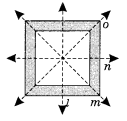(b) In this figure, there are four symmetric lines p, q, r and s.(c) In this shape, u, v , w and x are four lines of symmetry.(d) In this shape only m is the line of symmetry.(e) Here, a, b, c, d, e and fare six lines of symmetry.(f) In this figure l, m, n, o, p and q are six lines of symmetry.(g) This figure has no lines of symmetry.(h) This figure has no lines of symmetry.(i) This figure has five lines of symmetry.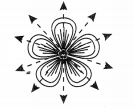Ex 13.2 Class 6 Maths Question 2.
Copy the triangle in each of the figures on squared paper. In each case, draw the line(s) of symmetry, if any and identify the type of triangle. (Some of you may like to trace the figures and try paper-folding first!)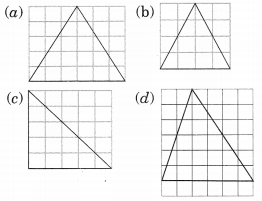Solution:
(a) It is an isosceles triangle having one symmetric line.(b) This figure is an isosceles triangle having only one symmetric line.(c) It is an isosceles right angled triangle which has only one symmetric line.(d) It is a scalene triangle. It has no symmetric line.Ex 13.2 Class 6 Maths Question 3.
Complete the following table.

 Shape Rough figure Number of lines of symmetry Equilateral triangle3 Square Rectangle Isosceles triangle Rhombus Circle

Solution:
Completed table:

 Shape Rough figure Number of lines of symmetry (a) Equilateral triangle3 (b) Square4 (c) Rectangle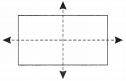2 (d) Isosceles triangle1 (e) Rhombus2 (f) CircleInfinite

Ex 13.2 Class 6 Maths Question 4.
Can you draw a triangle which has
(a) exactly one line of symmetry?
(b) exactly two lines of symmetry?
(c) exactly three lines of symmetry?
(d) no lings of symmetry?
Sketch a rough figure in each case.
Solution:
(a) Yes, Isosceles right angled triangle has exactly one line of symmetry.(b) No, we cannot draw any triangle with two symmetric lines.
(c) Yes, equilateral triangle has three lines of symmetry.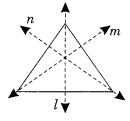(d) Yes, Scalene triangle has no lines of symmetryEx 13.2 Class 6 Maths Question 5.
On a squared paper, sketch the following:
(а) A triangle with a horizontal line of symmetry but no vertical line of symmetry.
(b) A quadrilateral with both horizontal and vertical lines of symmetry.

(c) A quadrilateral with a horizontal line of symmetry but no vertical line of symmetry.
(d) A hexagon with exactly two lines of symmetry.
(e) A hexagon with six lines of symmetry. (Hint: It will be helpful if you first draw the lines of symmetry and then complete the figures)
Solution:
(a) The figure shows an isosceles triangle with horizontal line of symmetry.(b) Rectangle (quadrilateral) shows both the horizontal and vertical lines of symmetry.(c) Trapezium (quadrilateral) shows the horizontal but no vertical line of symmetry.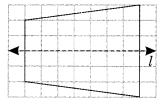(d) The hexagon drawn below shows only two lines of symmetry.(e) The regular hexagon shows the six lines of symmetry.Ex 13.2 Class 6 Maths Question 6.
Trace each figure and draw the lines of symmetry, if any.Solution:
(a) The given figure has no line of symmetry as it is not symmetrical.(b) The given figure has two lines of symmetry.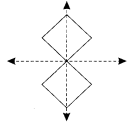(c) The given figure has four lines of symmetry.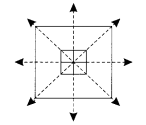(d) The given figure has two lines of symmetry.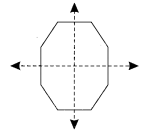(e) This figure has only one horizontal line of symmetry.(f) The given figure has two lines of symmetry.Ex 13.2 Class 6 Maths Question 7.
Consider the letters of English alphabets A to Z. List among them the letters which have
(a) vertical lines of symmetry, (like A)
(b) horizontal lines of symmetry (like B)
(c) no lines of symmetry, (like Q)
Solution:
(a) The following letters have vertical lines of symmetry:
A, H, I, M, O, T, U, V, W, X, and Y
(b) The following letters have horizontal lines of symmetry:
B, C, D, E, H, I, K, O and X.
(c) The following letters have no lines of symmetry:
F, G, J, L, N, P, Q, R, S and Z.

Ex 13.2 Class 6 Maths Question 8.
Given here are figures of a few folded sheets and designs drawn about the fold. In each case, draw a rough diagram of the complete figure that would be seen when the design is cut off.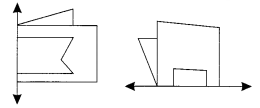Solution:
The given figures will be seen as follows when they are completed.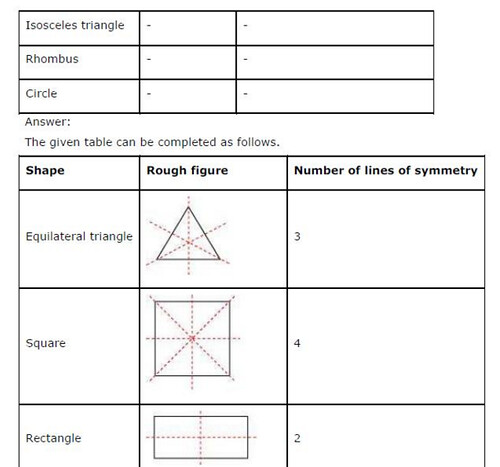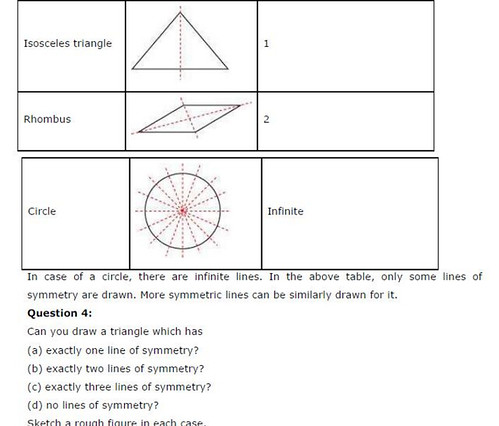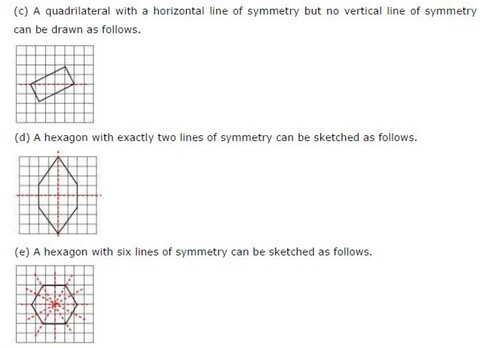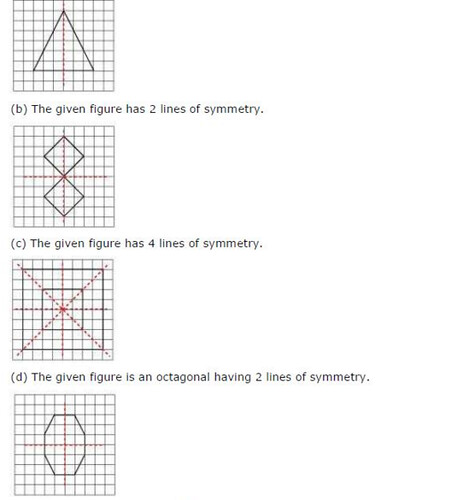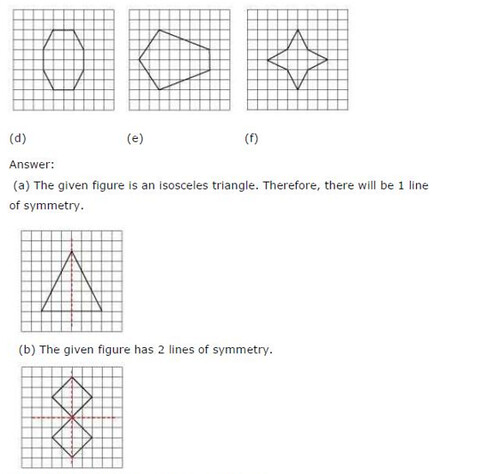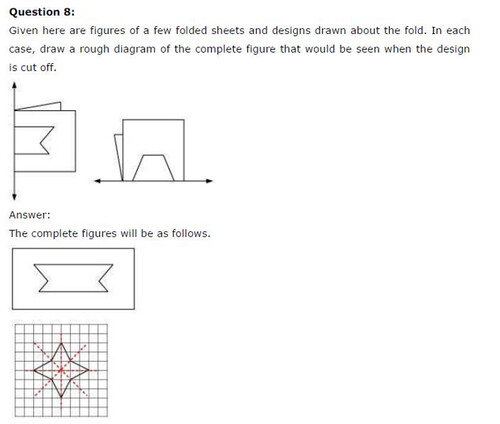## SabDekho

The Complete Educational Website